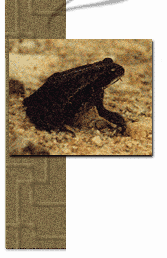Home Teachers | Mentors | Discussions | Research | Find
 Amphibian Biomonitoring Guided Research: Data AnalysisCreating  the Context   Home   Research Focus   Background Info   Research Methodology   Data Submission   Results of Study   Data Analysis   Conclusion   Further Research Guided Research   Research Question   Background Info   Research Methodology   Data Submission   Results of Study   Data Analysis   Conclusion   Further Research   Research Values Student Research   Publish   View Sample Analysis            Sample Data                                            ;      Analysis Blanchards Cricket Frog - 15                       Mean = (15 + 5 + 8 + 3 + 6)/5 = 7.4 Gray Treefrog                -  5                         Species Richness = total number of                                      &n bsp;                                                            species found American Toad              -  8                                         &n bsp;           = 5 Northern Spring Peeper -  3                          Species Diversity = 0.74 Southern Leopard Frog  -  6                                     (as shown below) Calculating diversity using the Simpson Diversity Index Calculate the proportion of the total number of amphibians of each species (Pi). Proportion species A (Pa) = number of species A/Total number of amphibians Proportion species B(Pb) = number of species B/Total number of amphibians To calculate the Simpson Diversity Index = 1 - sum(Pi)2          (meaning add the proportion of each squared species and take it from 1) Using the example above, you have 15 cricket frogs, 5 gray treefrogs, 8 american toads, 3 spring peepers, and 6 leopard frogs. You would calculate: Diversity Index = 1- ((15/37)2 + (5/37)2 + (8/37)2 + (3/37)2 + (6/37)2) Diversity Index = .74 This index ranges from zero to one and is literally a measure of the probability that two amphibianss taken at random from the sample are different species. A number close to zero means low diversity and it is likely you will get the same species of amphibian and a number close to one means high diversity.
 1999, KanCRN Collaborative Research Network